# Constructing minimal models for complex system dynamics

## Abstract

One of the strengths of statistical physics is the ability to reduce macroscopic observations into microscopic models, offering a mechanistic description of a system’s dynamics. This paradigm, rooted in Boltzmann’s gas theory, has found applications from magnetic phenomena to subcellular processes and epidemic spreading. Yet, each of these advances were the result of decades of meticulous model building and validation, which are impossible to replicate in most complex biological, social or technological systems that lack accurate microscopic models. Here we develop a method to infer the microscopic dynamics of a complex system from observations of its response to external perturbations, allowing us to construct the most general class of nonlinear pairwise dynamics that are guaranteed to recover the observed behaviour. The result, which we test against both numerical and empirical data, is an effective dynamic model that can predict the system’s behaviour and provide crucial insights into its inner workings.

## Introduction

Despite the marked advances in mapping social, biological and technological networks1,2,3,4,5, our ability to predict and manipulate their behaviour is currently limited due to the absence of accurate microscopic models of their interaction dynamics. Indeed, the observed behaviour of complex systems is governed by the interplay between their topology and their dynamics6,7, prompting us to develop reliable methodologies for the construction of dynamic models for complex systems. The problem is that unlike physical systems, where the interactions are described in terms of a set of fundamental rules, such as the laws of electromagnetism driving the microscopic interactions between particles, for most complex systems such rules remain to be discovered, limiting our ability to rely on a theoretical understanding of the system’s components when constructing the appropriate model. Here we develop a methodology to construct dynamic models directly from empirical observations, assuming minimal a priori knowledge about the system. Our goal is to directly translate these observations into a mechanistic model that accurately captures the interactions between the system’s components, ultimately providing the system’s equation of motion. Such models can provide both theoretical insights into the inner workings of the system and predictive power on its expected behaviour.

Consider a complex system with N components (nodes), whose activities xi(t) (i=1,…,N) are driven by ordinary differential equations of the form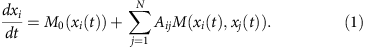Here M0(xi(t)) describes the self-dynamics of each component and M(xi(t), xj(t)) captures the impact of i’s neighbour j on the state of i. The adjacency matrix, Aij, describes which components interact. With the appropriate choice of the nonlinear M0(xi(t)) and M(xi(t), xj(t)), equation (1) has been used before to describe the dynamics of a wide range of complex systems, like metabolic networks8,9, the spread of infectious disease on a social network10,11 or interspecies interactions in ecological systems12, to mention only a few. Furthermore, for many biological9,13,14 technological15 or social10,11,16 systems the interaction term factorizes as M(xi(t), xj(t))=M1(xi(t))M2(xj(t)), in which case the system’s dynamics is uniquely characterized by three independent functions, together defining the system’s model as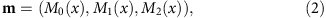a point in the model space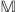(Fig. 1a,b). For systems of unknown microscopic dynamics, the challenge is to infer the appropriate model by identifying M0(x), M1(x) and M2(x) that accurately describe the system’s observable behaviour. (The treatment of more general systems, in which M(xi, xj) cannot be factorized, is discussed in Supplementary Note 5).

Traditionally, uncovering m involves three steps: (i) observation of the system’s macroscopic behaviour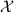(ref. 17); (ii) inference of the microscopic model, m, from; (iii) validation, showing that m can predict the observed behaviour. While this program has been successfully carried out for some well studied systems, the three steps above are difficult to replicate for complex systems: for instance, we lack general guidelines to choose the observationand a methodology to reliably translate it into a microscopic model. Hence steps (i) and (ii) require either an intuitive leap to guess the right observation and its inferred m, or exogenous knowledge, such as a mechanistic understanding of the nature of the interactions between the system’s components, knowledge we currently lack for many complex systems. Moreover, once m is found, step (iii) is insufficient to verify its uniqueness. Indeed, the inferred m could be one of a family of potential models that predict, which, absent any additional knowledge or observations, are all equally likely candidates for the system. This implies that rather than a specific point in the model space, what the observationtruly allows us to infer is a broader subspace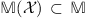, comprising all models m that can be validated against. A model not included in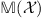can be ruled out by, however, all models that are included in this subspace are equally likely candidates andalone cannot be used to settle between them. Hence our goal is to develop a general method to infer, relying on minimal a priori knowledge of the structure of M0(x), M1(x) and M2(x).

Our method provides a systematic formalism to treat all steps (i)–(iii) above for constructing m directly from empirical data. As our input observationwe use the system’s response to external perturbations. This represents a common empirical exploration of complex systems, such as genetic perturbations in biology18,19, monitoring the impact of local failures in technological systems20 or tracking the spread of information in social networks21,22. Our key result is linking the observed system response to the leading terms of m, providing a direct formulation by which to translateinto an equation of the form (1). The inferred equation, reverse engineered directly from the data, does not provide a specific model m, but rather, defines the exact boundaries of, providing the most general class of dynamics that can be used to describe the observed system in light of.

## Results

### From observation to inference and validation

To infer m we express its components in terms of a Hahn series23 as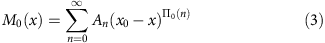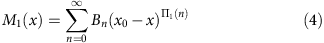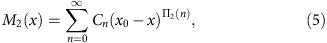which is a generalization of the Taylor expansion to include both negative and real powers. The powers Πi(n) represent a well-ordered set in ascending order with n, namely Πi(0) represents the leading power in the expansion of Mi(x) around x0, Πi(1)>Πi(0) is the next power and so on. For certain systems the functional form of (3)–(5) is known, and the challenge is to infer the specific coefficients An, Bn and Cn, which capture the model parameters, such as the rate constants in (1; refs 24, 25, 26). In contrast, here we consider systems whose microscopic model itself is unknown. Our goal is thus to uncover the functional form of (3)–(5), which is captured by the powers Πi(n) that participate in the expansion. Hence we do not focus here on distinctions such as M2(x)=x2 or M2(x)=2x2, a distinction regarding the coefficient Cn, which describes the rate at which the interaction occurs. Rather, our focus here is on distinguishing between M2(x)x2 versus, say, M2(x)1–x−2, as the different powers expressed in the expansion, Π2(n), capture different interaction mechanisms, and hence provide an insight into the fundamental characteristics of the system’s dynamics.

To infer the leading powers of (3)–(5) we link them to a set of experimentally accessible observables, related to the system’s response to external perturbations. These observables, in turn, allow us to recover the structure of (3)–(5), reverse engineering the dynamics (1) to its leading terms. To conduct the observation, first we subject the nodes to external perturbations: this entails permanently perturbing the steady-state activity, xj of node j, and capturing the response of all other node activities xi(t). This could be achieved either through a controlled experiment, as frequently done in genetic perturbations18, or by monitoring natural perturbations, like observing the spread of ideas or memes in a social network21,22, or cascading failures in technological systems20. The impact of these perturbations is captured by two quantities (observations):

Transient response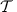. The temporal dynamics following a permanent perturbation is characterized by the time-dependent relaxation from the original steady state, xi, to the perturbed steady-state xi(t→∞)=xi+dxi. By linearizing (1) around the steady state we find (Supplementary Note 1)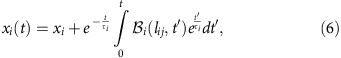where τi is node i’s relaxation time and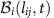accounts for the propagation of the permanent perturbation along a distance lij from the source j to the observed node i.

Asymptotic response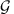. After relaxation (tmax(τi)), the system reaches a new, permanently perturbed state, captured by the response matrix6,7,19,27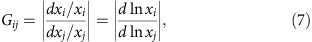which quantifies the response of node i to j’s perturbation (Supplementary Note 2). By extracting a set of empirically observable exponents from the transient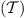and the asymptotic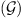responses, we can link xi(t) (6) and Gij (7) to m (2) via (Supplementary Note 3)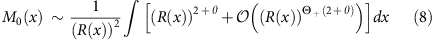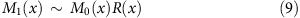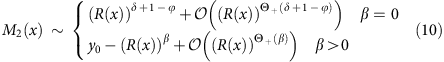where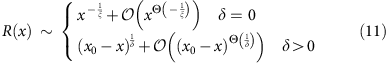and x0 and y0 are arbitrary constants.

Equations (8)–(11), , , take a set of observables (exponents) extracted from the two observations,(6) and(7), as input (see Table 1), and provide the leading powers in the expansions (3)–(5) as output. Fromwe can directly extract two exponents (Supplementary Note 1): (i) θ captures the dependence of the relaxation time on the node’s degree ki, which follows the scaling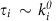and (ii) ξ describes the steady-state activity, which can either follow (Supplementary Note 1)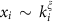(scaling) or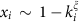(saturation). Fromwe can extract (Supplementary Note 2, ref. 7): (i) ϕ, capturing i’s impact on its local neighbourhood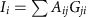, which scales as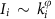; (ii) δ, capturing i’s stability against perturbations in its vicinity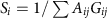, which follows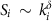; and (iii) β, the dissipation rate that captures the behaviour of the distance-dependent propagation function, Γ(l). This function describes the aggregated response of all nodes at distance l from a perturbation. For a system following (1) we have Γ(l)=eβαl, where α=ln(〈k2〉/〈k〉−1) is the expansion rate of the network. Note that to measure the observables used for the reconstruction (8)–(11) we must have access to the degrees ki of all the nodes, requiring us to know the network topology Aij. Later we show that even without access to Aij, a partial reconstruction of m is still possible.

The inference presented in (8)–(11) provides only the leading terms of M0(x), M1(x) and M2(x). This leaves a degree of freedom to add additional terms, as denoted by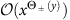, which involves all the terms of order higher (Θ+(y)) or lower (Θ(y)) than xy. Finally, in (11) we used the unsigned Θ(y)=Θsign(y)(y), which allows the inclusion of higher (lower) powers for y>0 (y<0). Hence by measuring the three characteristic exponents of(δ, ϕ and β), together with the two characteristic exponents of(θ and ξ), we can reconstruct m to its leading order terms, allowing us to write an effective continuum equation (1) for the system.

Equations (8)–(11), , , represent our main result, offering a formalism to systematically reconstruct the dynamical equation governing a complex system. As expected, they do not point to a specific model, m, but narrow down the spaceof all the potential models into the minimal subspace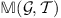of the models that are consistent with observationsand(Fig. 1e). This subspace is robust to adding higher order terms and to parameter selection (that is, rate constants). Therefore it defines a minimal model, capturing the essential aspects of the mechanisms underlying the system’s interactions. Most importantly, our formalism guarantees that all models included inwill successfully validate againstand, and conversely all models for which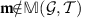will not be consistent with observationsand. Hence if and only if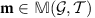can m be validated against the experimentally measured ξ and θ (), and δ, ϕ and β ().

### Inferring the dynamics of a model system

To illustrate the predictive power of the developed formalism we first apply it to gene regulation, where the interaction is captured by a Hill function as (refs 13, 14, 28)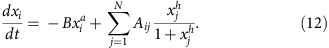Here B is a rate constant, a represents the level of self-regulation and the Hill coefficient, h, describes the level of cooperativity in gene regulation. We set B=1, a=1/2 and h=1/3, hence the real dynamics (12) is captured by the model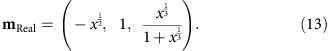Next we assume that mReal is unknown and preform an in silico reconstruction using (8)–(10). To observeandwe perturb each node around the steady state and numerically measure xi(t) (6) and Gij (7) (Supplementary Note 4). Forwe find ξ=2.0±0.02 and θ=1.0±0.01 (Fig. 2a,b); forwe observe δ=0, ϕ=0.33±0.01 and β=0.67±0.01 (Fig. 2c–e). Therefore (8)–(10) predict (Supplementary Note 4)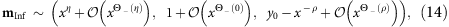where η=0.50±0.01 and ρ=0.33±0.03. Hence the reverse engineered dynamical model for the system has the form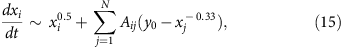where we only included the leading terms of (14). Equation (15) accurately recovers the self-dynamics and the interaction terms to leading order (Fig. 2f–h). Indeed, expanding M2(x) in (13) for large x leads to the inferred M2(x) in (15). Hence, the inferred M2(x) not only captures the qualitative form of the Hill function, that is, the saturation of the interaction term for large x (ref. 28), but also the precise form of that saturation as 1−x−1/3+…. This demonstrates that our formalism can correctly reverse engineer the system’s microscopic dynamics directly from observingand.

The inferred m can be also used to predict a broader range of macroscopic functions of direct experimental interest. Consider for example the probability density P(G) that a response term Gij is between G and G+dG. We can show that P(G)Gν, where ν=(β+2)/(β+1) (ref. 7). Another quantity frequently observed in biological18, social21,22,29 and technological20,30,31 networks is the cascade size distribution, P(C), representing the probability that exactly C nodes exhibit a significant response (above a threshold) to a perturbation. This distribution is driven by the degree distribution through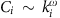, where ω=(β+ϕ)/(β+1), predicting P(C) through P(C)P(kω=C) (ref. 7). Finally, the incoming cascade of node i, defined as the group of all nodes whose perturbation impacted i above a threshold, can be shown to follow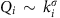where σ=(βδ)/(β+1). Hence, by recovering β, ϕ and δ, the inferred m is guaranteed to also predict P(G), P(C) and P(Q) (Supplementary Note 2).

### Inferring the dynamics of empirical systems

In experimental settings, we rarely have access to all the components ofand. In some cases we may lack access to the temporal dynamics, unable to measure ξ and θ; in others we lack a map of the underlying network, missing ki. Fortunately, the three additional exponents ν, ω and σ, associated with the cascades and with the distribution of terms in Gij (Table 1), provide an excess of experimentally accessible quantities to arrive at the original exponents required for the reconstruction (8)–(10). This redundancy enables us to obtain insights from partial observations as well. We illustrate this by inferring the dynamical model for two systems that span rather different domains of inquiry: cell biology and human activity.

Reverse-engineering subcellular dynamics. We demonstrate the utility of our formalism using results obtained from high-throughput gene perturbation experiments for S. cerevisiae. Here Gij measures the change in the expression levels of 6,222 yeast genes induced by 55 individual genetic perturbations32. As the data set is not time resolved, we lack access to. We also lack an accurate map of the underlying regulatory/protein interaction network, hence we cannot directly measure δ, ϕ and β. Our method offers valuable insights even under these rather limiting circumstances. First, we measure the distribution of terms in Gij, which we find to follow P(G)Gν where ν=2.0±0.1 (Fig. 3a). Using Table 1 this translates to β=0. Next we measure the distribution P(Q) of the incoming cascades of all the nodes (Fig. 3b). Its bounded nature implies that it is disconnected from the degree heterogeneity (P(k))33,34, possible only if σ=0, which in turn provides δ=0 (Table 1). Hence using (8)–(10) we obtain (Supplementary Note 6)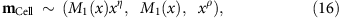where, for simplicity, we once again omitted the higher order terms. Equation (16) predicts a family of potential models, with an arbitrary M1(x) and η and ρ, degrees of freedom originating in our partial coverage of the functions used as input in (8)–(10). Indeed, it is expected than the less specific is the observation, the broader are the limits of the inferred subspace. Despite these degrees of freedom, (16) offers crucial insight into the biological mechanisms that underlie the observed dynamics, helping us distinguish between the two classes of dynamical processes that potentially drive the expression patterns of genes in the studied experiment. The first process is regulatory interactions (RIs), the mutual activation/inhibition of genes, in which the interaction term has the form of a switch-like function, for example, Hill function (12), saturating as M2(x→∞)→1 (M2(x→∞)→0), to describe the activation (inhibition)13,14 (Figs 2h and 3c). A competing process is protein–protein interactions (PPIs), a biochemical mechanism in which proteins physically bind to each other. PPIs are expressed in (1) through mass action kinetics by non-saturating polynomial terms. Indeed, according to the law of mass action a physical binding interaction μA+ρBAB contributes a term of the form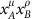to the relevant equations in (1) (refs 9, 15, 35, 36). Hence the polynomial (non-saturating) form of M0(x) and M2(x) in (16) indicates that in this experiment the system’s dynamics is dominated by biochemical interactions, such as protein binding, degradation and dimerization, rather than genetic regulation.

To directly test this prediction we used validated lists of 2,930 PPIs (ref. 34) and 1,079 RIs37. It is expected that a large response Gij indicates an increased probability of finding a direct i, j link. Hence we can use Gij to predict the already known PPI/RI links. The standard measures to evaluate such predictions are the areas under the receiver operating curve (AUROC) and under the precision recall curve (AUPR)38 (Supplementary Note 6). Measuring these curves (Fig. 3d,e), we find that in this experiment Gij is indeed more predictive of PPIs than of RIs: for PPIs we obtain AUROC=0.580 (P value 0.03, Supplementary Note 6) and AUPR=1.8 × 10−3 (P value 0.07), while for RIs we find AUROC=0.504, and AUPR=1.4 × 10−3, both not significantly better than random (P value 1). Hence, even though cellular dynamics is driven by a combinations of both RIs and PPIs, in this experiment Gij emphasizes PPIs significantly more than the RIs, supporting our conclusion that PPIs, driven by biochemistry, offer the dominant contribution to the experimentally observed Gij, in agreement with the inferred (16). This finding is, in fact, consistent with other studies indicating that PPIs play a significant role in shaping the profile of expression data39,40,41. Therefore the strength of our formalism is not only its ability to reconstruct the continuum model (16), but also its ability to detect the dominance of PPIs in this experiment using only the observed data Gij.

Reverse-engineering human dynamics. While building continuum models for biological systems has a long tradition, the diversity of human interaction has lead to a paucity of continuum models capturing human dynamics42,43. Here we rely on a data set that captures 6 × 104 exchanges between 1,899 users of an online instant messaging service (UCIonline) during a 7 month period (ref. 44). This allowed us to construct Aij by linking each user pair that exchanged at least one message during the documented period. As here we cannot conduct a controlled perturbation experiment, we rely on proxies that capture quantities associated with xi in (6) and Gij in (7). First we measure the number of messages sent by user i during a 3 hours interval, xi(t), to obtain its time-dependent activity. We take xi=<xi(t)> as a proxy for i’s steady-state activity and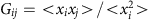as a proxy for (7) (Supplementary Note 7). We find ξ=1.23±0.03, δ=0 and ν=1.60±0.03, predicting β=0.67 (Table 1, Fig. 4a–c). Hence equations (8)–(10), , predict that the continuum model capturing the dynamics of this social system has the form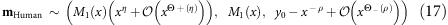where η=0.81, ρ=0.54 and M1(x) is an arbitrary function.

To understand the inferred dynamics, we expand M1(xi) as in (4) and take its leading power to be μ, namely Π1(0)=μ. Hence the self-dynamic term in (17) becomes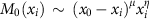. Note that this term provides the node’s dynamics in isolation, as in the absence of interacting partners equation (1) reduces to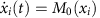. Here as we describe the message exchanges between linked individuals, an isolated node should become inactive, xi(t→∞)=0, a condition satisfied if we set x0=0. Hence, taking only the leading terms of mHuman we obtain the continuum equation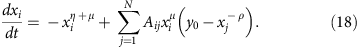To evaluate μ we consider the workload Wi of pending messages that have to be sent or replied and its impact on i’s activity xi. When the workload Wi is large, i experiences a significant pressure to respond, increasing its activity xi. Yet Wi decreases with every email i sends, hence a highly active i will rapidly decrease its workload and activity. Equation (18) predicts that the workload should increase with i’s activity as (Supplementary Note 7)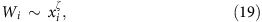where ζ=2–ημ. To test the validity of this prediction we measured the incoming messages of all nodes as a proxy for their workload, finding that the predicted scaling (19) holds for over three orders of magnitude with ζ=0.73±0.02, predicting μ=0.46 (Fig. 4d). The second term on the right hand side of equation (18) describes the impact of the neighbours’ activity xj on xi. An active neighbour j increases i’s activity, prompting it to reply or forward its incoming messages. The saturating nature of M2(x), however, indicates that a neighbour’s impact is bounded, reaching a maximum of y0. This agrees with our expectation that even an extremely active neighbour cannot drive its contacts to be active beyond their maximal capacity.

To validate (18) we used an independent data set, recording 3 × 105 email exchanges between 2,688 users during a 6-month period (Epoch) (ref. 45). The exponents extracted from this data set are very close to those obtained from UCIonline: ξ=1.26±0.04, δ=0 and ν=1.57±0.05 (Fig. 4f–h). The workload (19), however, has ζ=0.35±0.1, which significantly differs from that of UCIonline (Fig. 4i). Reverse engineering the dynamics from these exponents leads to (18), with η=0.79, μ=0.86 and ρ=0.60. This striking agreement between the structure of the two equations inferred from two independent data sets, indicates that the reverse engineered (18) captures the fundamental dynamical characteristics of human communications. The only notable difference between the two inferred models is in the value of μ, which is higher in Epoch than in UCIonline. This parameter characterizes the correlation between a node’s activity and its tendency to respond to incoming messages (Supplementary Note 7). Hence the greater value of μ in Epoch suggests that the propensity to respond to incoming communications is more strongly dependent on the activity in email communication than in instant messaging. To test if this is indeed the case we measured the responsiveness, Ri, of all nodes, defined as the average ratio between the incoming and outgoing traffic between i and its interacting partners. As μ is greater for Epoch than UCIonline, we expect a stronger dependency in Epoch between Ri and xi than in UCIonline. Indeed, as shown in Fig. 4e,j this prediction is valid: the scaling of Ri with xi, is greater for Epoch (γ=0.37) than for UCIonline (γ=0.14). This dynamical distinction, correctly predicted by the reverse engineered equations, provides independent support for the predictive power of our formalism.

The empirical results presented above demonstrate the practical applicability of our methodology, which is a result of the robust nature of its underlying observables. Indeed, characteristic exponents and scaling laws, the basis of our reverse-engineering formalism, are often universal46, and hence unaffected by the microscopic details of the system’s topology and dynamics7. This allows us to reliably measure the observables even for real systems, which rarely satisfy all of the model assumptions, for example, they are subject to the effect of noise, both in their dynamics as well as in their topology. For example, many real networks feature degree correlations, which, strictly speaking, violate our formalism’s predictions (Supplementary Note 1). Fortunately, the observed exponents are rather insensitive to such microscopic discrepancies, and can be accurately extracted from both model and empirical systems, even in the presence of degree correlations. To exemplify this we simulated the predicted human dynamics (18) on the empirical network of Email Epoch. Even though this network features rather strong degree correlations, we show in Supplementary Note 7 that the measured obsevables, that is, the exponents ξ, δ and ν, can be accurately fitted by the predictions of our formalism.

## Discussion

In summary, the technological and experimental advances of recent years have offered a wealth of data, capturing the detailed node-level dynamics of biological, social and technological systems. It is difficult, however, to extract predictive power of these systems without a mechanistic model. Such models are rare for complex systems, however. Here we address this challenge as a reverse-engineering problem, showing that we can use the data to peek into the inner mechanisms of the system, providing an analytical microscope into the dynamics of a complex system. We tested our formalism under rather strict conditions, inferring m from scratch, relying only on the system’s macroscopic behaviour (and). A more realistic scenario, however, is to use the proposed method in conjunction with some prior knowledge about the system’s microscopic dynamics. Often we seek a resolution between two or more competing models, as was the case in our inference of the cellular dynamics. If the two candidate models have a different functional form in (1), they will occupy distinct subspaces in, and the more likely of the two can be decisively determined (Fig. 3c). In other cases, the inference can be supported by some a priori knowledge pertaining to the system’s behaviour. For instance, in human dynamics we postulated, based on the nature of the observed system, that isolated individuals should become inactive. Coupled with our formalism (8)–(10), this allowed us to complete the reconstruction of (18). Other practical considerations in reverse engineering are addressed in Supplementary Note 7.

Finally, the fact that our formalism infers a subspace, rather than a specific model m provides us with exact bounds on the predictive power of an observation. Indeed, it tells us that our observation,, provides us with theoretical grounds to select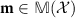over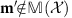. At the same time, however, our formalism shows thatcannot be used to discriminate between any set of models within, by that marking the theoretical limits on the specificity of the inferred model.

How to cite this article: Barzel, B. et al. Constructing minimal models for complex system dynamics. Nat. Commun. 6:7186 doi: 10.1038/ncomms8186 (2015).

## References

1. 1

Strogatz, S. H. Exploring complex networks. Nature 410, 268–276 (2001).

2. 2

Drogovtsev, S. N. & Mendez, J. F. F. Evolution of Networks: From Biological Nets to the Internet and WWW. Oxford Univ. Press (2003).

3. 3

Caldarelli, G. Scale-free Networks: Complex Webs in Nature and Technology Oxford Univ. Press (2007).

4. 4

Helbing D., Jost J., Kantz H. eds. Networks and complexity. Netw. Heterog. Media 3, 185–411AIMS, Springfield (2008).

5. 5

Newman, M. E. J. Networks—An Introduction Oxford Univ. Press (2010).

6. 6

Barzel, B. & Biham, O. Quantifying the connectivity of a network: the network correlation function method. Phys. Rev. E 80, 046104 (2009).

7. 7

Barzel, B. & Barabási, A.-L. Universality in network dynamics. Nat. Phys. 9, 673–681 (2013).

8. 8

Murray, J. D. Mathematical Biology Springer (1989).

9. 9

Voit, E. O. Computational Analysis of Biochemical Systems Cambridge Univ. Press (2000).

10. 10

Hufnagel, L., Brockmann, D. & Geisel, T. Forecast and control of epidemics in a globalized world. Proc. Natl Acad. Sci. USA 101, 15124 (2004).

11. 11

Dodds, P. S. & Watts, D. J. A generalized model of social and biological contagion. J. Theor. Biol. 232, 587–604 (2005).

12. 12

Károlyi, G., Pãntek, Á., Scheuring, I., Tél, T. & Toroczkai, Z. Chaotic flow: the physics of species coexistence. Proc. Natl Acad. Sci. USA 97, 13661 (2000).

13. 13

Alon, U. An Introduction to Systems Biology: Design Principles of Biological Circuits Chapman & Hall (2006).

14. 14

Karlebach, G. & Shamir, R. Modelling and analysis of gene regulatory networks. Nat. Rev. 9, 770–780 (2008).

15. 15

Gardiner, C. W. Handbook of Stochastic Methods Springer-Verlag (2004).

16. 16

Pastor-Satorras, R. & Vespignani, A. Epidemic spreading in scale-free networks. Phys. Rev. Lett. 86, 3200–3203 (2001).

17. 17

Liu, Y.-Y., Slotine, J.-J. & Barabási, A.-L. Observability of complex systems. Proc. Natl Acad. Sci. USA 110, 2460–2465 (2013).

18. 18

Kauffman, S. The ensemble approach to understand genetic regulatory networks. Physica A 340, 733–740 (2004).

19. 19

Maslov, S. & Ispolatov, I. Propagation of large concentration changes in reversible protein-binding networks. Proc. Natl Acad. Sci. USA 104, 13655 (2007).

20. 20

Dobson, I., Carreras, B. A., Lynch, V. E. & Newman, D. E. Complex systems analysis of series of blackouts: cascading failure, critical points, and self-organization. Chaos 17, 026103 (2007).

21. 21

Leskovec, J., Singh, A. & Kleinberg, J. Patterns of influence in a recommendation network. Lect. Notes Comput. Sci. 3918, 380 (2006).

22. 22

Leskovec, J., Mcglohon, M., Faloutsos, C., Glance, N. & Hurst, M. Patterns of cascading behavior in large blog graphs. Proc. SIAM Inter. Conf. Data Mining 551–556 (2007).

23. 23

Schmetterer L., Sigmund K. (Eds.) Hans Hahn Gesammelte Abhandlungen Band 1/Hans Hahn Collected Works Volume 1 Springer (1995).

24. 24

Walter, É. & Proznato, L. Identification of Parametric Models From Experimental Data Masson (1997).

25. 25

Jin, G., Sain, M. K., Pham, K. D., Spencer, B. F. Jr. & Ramallo, J. C. Modeling MR-dampers: a nonlinear blackbox approach. In Proc. Am. Control Conf June 25-27, 429–434Springer-Verlag, IEEE (2001).

26. 26

Nielsen, H. A. & Madsen, H. Modelling the heat consumption in district heating systems using a grey-box approach. Energ Buildings 38, 63–71 (2006).

27. 27

Barzel, B. & Barabási, A.-L. Network link prediction by global silencing of indirect correlations. Nat. Biotechnol. 31, 720–725 (2013).

28. 28

Gómez-Gardeñes, J., Moreno, Y. & Floría, L. M. Michaelis-Menten dynamics in complex heterogeneous networks. Physica A 352, 265–281 (2005).

29. 29

Kitsak, M. et al. Identification of influential spreaders in complex networks. Nat. Phys. 6, 888–893 (2010).

30. 30

Crucitti, P., Latora, V. & Marchiori, M. Model for cascading failures in complex networks. Phys. Rev. E 69, 045104 (2004).

31. 31

Buldyrev, S. V., Parshani, R., Paul, G., Stanley, H. E. & Havlin, S. Catastrophic cascade of failures in interdependent networks. Nature 464, 1025–1028 (2010).

32. 32

Chua, G. et al. Identifying transcription factor functions and targets by phenotypic activation. Proc. Natl Acad. Sci. USA 103, 12045 (2006).

33. 33

Guelzim, N., Bottani, S., Bourgine, P. & Képès., F. Topological and causal structure of the yeast transcriptional regulatory network. Nat. Genet. 31, 60–63 (2002).

34. 34

Yu, H. et al. High-quality binary protein interaction map of the yeast interactome network. Science 322, 104–110 (2008).

35. 35

Barzel, B. & Biham, O. Binomial moment equations for stochastic reaction systems. Phys. Rev. Lett. 106, 150602 (2011).

36. 36

Barzel, B. & Biham, O. Stochastic analysis of complex reaction networks using binomial moment equations. Phys. Rev. E 86, 031126 (2012).

37. 37

Milo, R. et al. Network motifs: Simple building blocks of complex networks. Science 298, 824–827 (2002).

38. 38

Marbach, D. et al. Wisdom of crowds for robust gene network inference. Nat. Methods 9, 796–804 (2012).

39. 39

Ge, H., Liu, Z., Church, G. M. & Vidal, M. Correlation between transcriptome and interactome mapping data from Saccharomyces cerevisiae. Nat. Genet. 29, 482–486 (2001).

40. 40

Jansen, R., Greenbaum, D. & Gerstein, M. Relating whole-genome expression data with protein-protein interactions. Genome Res. 12, 37–46 (2002).

41. 41

Bhardwaj, N. & Lu, H. Correlation between gene expression profiles and protein-protein interactions within and across genomes. Bioinformatics 21, 2730–2738 (2005).

42. 42

Castellano, C., Fortunato, S. & Loreto, V. Statistical physics of social dynamics. Rev. Mod. Phys. 81, 591–646 (2009).

43. 43

Moretti, P., Liu, S., Castellano, C. & Pastor-Satorras, R. Mean-field analysis of the q-voter model on networks. J. Stat. Phys. 151, 113–130 (2013).

44. 44

Opsahl, T. & Panzarasa, P. Clustering in weighted networks. Social Networks 31, 155–163 (2009).

45. 45

Eckmann, J.-P., Moses, E. & Sergi, D. Entropy of dialogues creates coherent structures in e-mail traffic. Proc. Natl Acad. Sci. USA 101, 14333 (2004).

46. 46

Wilson, K. G. The renormalization group: critical phenomena and the Kondo problem. Rev. Mod. Phys. 47, 773–840 (1975).

## Acknowledgements

This work was supported by the Templeton Foundation: Mathematical and Physical Sciences grant no. PFI-777; Army Research Laboratories (ARL) Network Science (NS) Collaborative Technology Alliance (CTA) grant: ARL NS-CTA W911NF-09-2-0053; European Union grant no. FP7 317532 (MULTIPLEX).

## Author information

Authors

### Contributions

All authors designed the research and wrote the paper. B.B. analyzed the empirical data, and did the analytical and numerical calculations.

### Corresponding author

Correspondence to Yang-Yu Liu.

## Ethics declarations

### Competing interests

The authors declare no competing financial interests.

## Supplementary information

### Supplementary Information

Supplementary Figures 1-9, Supplementary Notes 1-7 and Supplementary References (PDF 433 kb)

## Rights and permissions

Reprints and Permissions

• ### DNF: A differential network flow method to identify rewiring drivers for gene regulatory networks

• Jiang Xie
• , Fuzhang Yang
• , Jiao Wang
• , Mathew Karikomi
• , Yiting Yin
• , Jiamin Sun
• , Tieqiao Wen
•  & Qing Nie

Neurocomputing (2020)

• ### Linear processes on complex networks

• Ivan Jokić
• , Piet Van Mieghem
•  & Yamir Moreno

Journal of Complex Networks (2020)

• ### Spatiotemporal signal propagation in complex networks

• Chittaranjan Hens
• , Uzi Harush
• , Simi Haber
• , Reuven Cohen
•  & Baruch Barzel

Nature Physics (2019)

• ### Dynamic interdependence and competition in multilayer networks

• Michael M. Danziger
• , Ivan Bonamassa
• , Stefano Boccaletti
•  & Shlomo Havlin

Nature Physics (2019)

• ### Effects of Population Dynamics on Establishment of a Restriction-Modification System in a Bacterial Host

• Stefan Graovac
• , Andjela Rodic
• , Magdalena Djordjevic
• , Konstantin Severinov
•  & Marko Djordjevic

Molecules (2019)